The GENMOD Procedure

Exact Logistic and Exact Poisson Regression

Subsections:

The theory of exact logistic regression, also called exact conditional logistic regression, is described in the section Exact Conditional Logistic Regression in Chapter 60: The LOGISTIC Procedure. The following discussion of exact Poisson regression, also called exact conditional Poisson regression, uses the notation given in that section.

Note that in exact logistic regression, the coefficients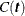are the number of possible response vectors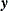that generate: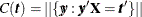. However, when performing an exact Poisson regression, this value is replaced by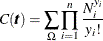where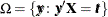andis the exponential of the offset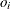for observation i. If an offset variable is not specified, then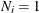.

The probability density function (PDF) for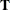is created by summing over all candidate sequencesthat generate an observable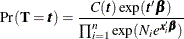However, the conditional likelihood of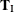given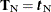has the same form as that for exact logistic regression.

For details about hypothesis testing and estimation, see the sections Hypothesis Tests and Inference for a Single Parameter in Chapter 60: The LOGISTIC Procedure. See the section Computational Resources for Exact Logistic Regression in Chapter 60: The LOGISTIC Procedure, for some computational notes about exact analyses.

In exact logistic binary regression, each componentofcan take a value of 0 or 1, so there are a finite number,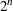, of candidatevectors to be considered. Since a Poisson-distributed response variable can take an infinite number of values, exact Poisson regression should evaluate an infinite number ofvectors. However, by identifying the maximum value ofto check,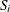, for each observation i, the number of candidatevectors to check is reduced to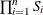. On a practical level, asbecomes large the probability of the Poisson random variable achieving this value drops to zero, socan be thought of as the point at which the value does not matter. You can provide these maxima by specifying either an OFFSET= variable,, or an EXACTMAX= variable,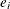, or you can let the algorithm choose a maximum for you. The way these two options interact to provide a maximum is described in the following list:

1. If an EXACTMAX= variable is specified, then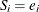.

2. If the EXACTMAX option is specified without a variable, or if neither the EXACTMAX= nor OFFSET= options are specified, then you must also condition out the intercept or you must specify the STRATA statement. If you are conditioning out the intercept, then everyhas an effective maximum of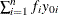, where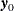is the observed response and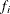is the frequency of the observation; this is the sufficient statistic for the intercept term. If you are performing a stratified analysis, these sums are computed within each stratum.

3. If an offset variable is specified and the EXACTMAX option is not specified (you are modeling proportions), thenmust be a positive integer, and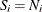is the maximum possible value for each observation in the experiment; for example, if you are counting the number of rats in a cage that acquire a disease, then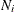is the number of rats in cage i.

OUTDIST= Output Data Set

The OUTDIST= data set contains every exact conditional distribution necessary to process the corresponding EXACT statement. For example, the following statements create one distribution for the x1 parameter and another for the x2 parameters, and produce the data set dist shown in Table 43.11:

data test;
input y x1 x2 count;
datalines;
0 0 0 1
1 0 0 1
0 1 1 2
1 1 1 1
1 0 2 3
1 1 2 1
1 2 0 3
1 2 1 2
1 2 2 1
;

proc genmod data=test exactonly;
class x2 / param=ref;
model y=x1 x2 / d=b;
exact x1 x2/ outdist=dist;
run;
proc print data=dist;
run;


Table 43.11: OUTDIST= Data Set

Obs

x1

x20

x21

Count

Score

Prob

1

.

0

0

3

5.81151

0.03333

2

.

0

1

15

1.66031

0.16667

3

.

0

2

9

3.12728

0.10000

4

.

1

0

15

1.46523

0.16667

5

.

1

1

18

0.21675

0.20000

6

.

1

2

6

4.58644

0.06667

7

.

2

0

19

1.61869

0.21111

8

.

2

1

2

3.27293

0.02222

9

.

3

0

3

6.27189

0.03333

10

2

.

.

6

3.03030

0.12000

11

3

.

.

12

0.75758

0.24000

12

4

.

.

11

0.00000

0.22000

13

5

.

.

18

0.75758

0.36000

14

6

.

.

3

3.03030

0.06000

The first nine observations in the dist data set contain an exact distribution for the parameters of the x2 effect (hence the values for the x1 parameter are missing), and the remaining five observations are for the x1 parameter. If a joint distribution was created, there would be observations with values for both the x1 and x2 parameters. For CLASS variables, the corresponding parameters in the dist data set are identified by concatenating the variable name with the appropriate classification level.

The data set contains the possible sufficient statistics of the parameters for the effects specified in the EXACT statement, and the Count variable contains the number of different responses that yield these statistics. In particular, there are six possible response vectorsfor which the dot product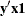was equal to 2, and for which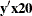,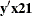, and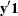were equal to their actual observed values (displayed in the "Sufficient Statistics" table).

Note: If you are performing an exact Poisson analysis, then the Count variable is replaced by a variable named Weight.

When hypothesis tests are performed on the parameters, the Prob variable contains the probability of obtaining that statistic (which is just the count divided by the total count), and the Score variable contains the score for that statistic.

The OUTDIST= data set can contain a different exact conditional distribution for each specified EXACT statement. For example, consider the following EXACT statements:


exact 'O1'   x1    /           outdist=o1;
exact 'OJ12' x1 x2 / jointonly outdist=oj12;
exact 'OA12' x1 x2 / joint     outdist=oa12;
exact 'OE12' x1 x2 / estimate  outdist=oe12;


The O1 statement outputs a single exact conditional distribution. The OJ12 statement outputs only the joint distribution for x1 and x2. The OA12 statement outputs three conditional distributions: one for x1, one for x2, and one jointly for x1 and x2. The OE12 statement outputs two conditional distributions: one for x1 and the other for x2. Data set oe12 contains both the x1 and x2 variables; the distribution for x1 has missing values in the x2 column while the distribution for x2 has missing values in the x1 column.